﻿ Diagonal Function of k-Lucas Polynomials

### Diagonal Function of k-Lucas Polynomials

Yogesh Kumar Gupta, V. H. Badshah, Mamta Singh, Kiran SisodiyaOPEN ACCESSPEER-REVIEWED

## Diagonal Function of k-Lucas Polynomials

Yogesh Kumar Gupta1,, V. H. Badshah1, Mamta Singh2, Kiran Sisodiya1

1School of Studies in Mathematics, Vikram University Ujjain, (M. P.) India

2Department of Mathematical Science and Computer applications, Bundelkhand University, Jhansi (U. P.) India

### Abstract

The Lucas polynomials are famous for possessing wonderful and amazing properties and identities. In this paper, Diagonal function of k-Lucas Polynomials is introduced and defined by Gn+1(x)=kxGn(x)+Gn-2,(x), n≥1. with G0(x)=2. and G1(x)=1 Some Lucas Polynomials, rising & descending diagonal function and generating matrix established and derived by standard methods.

• Gupta, Yogesh Kumar, et al. "Diagonal Function of k-Lucas Polynomials." Turkish Journal of Analysis and Number Theory 3.2 (2015): 49-52.
• Gupta, Y. K. , Badshah, V. H. , Singh, M. , & Sisodiya, K. (2015). Diagonal Function of k-Lucas Polynomials. Turkish Journal of Analysis and Number Theory, 3(2), 49-52.
• Gupta, Yogesh Kumar, V. H. Badshah, Mamta Singh, and Kiran Sisodiya. "Diagonal Function of k-Lucas Polynomials." Turkish Journal of Analysis and Number Theory 3, no. 2 (2015): 49-52.

 Import into BibTeX Import into EndNote Import into RefMan Import into RefWorks

### 1. Introduction

The sequence 1,1,2,3,5..., got its name as name as Fibonacci sequence by the Famous Mathematics Francois Edouard Lucas in 1876 .

Lucas also discovered a new Fibonacci like sequence with different initial condition call it, Lucas Sequence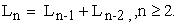with initial condition,In 1965 Hoggatt, V.E.  has defined Lucas polynomials by recurrence relation.where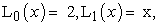(1.1)

The first few Lucas polynomials areIn this paper, we are using the pair of sequence {Gn} and {Pn} for which,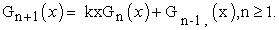(1.2)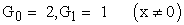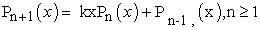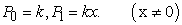(1.3)

where k is any positive integer. k= 0, 1, 2, 3...

Using the equation (1.1) and (1.2) and made a rising diagonal function and descending Diagonal Functions.

### 2. Sequence {Gn} and {Pn}

We have the pair of sequence {Gn} and {Pn} for which,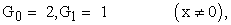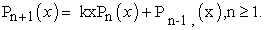The first few terms of the sequence {Gn} are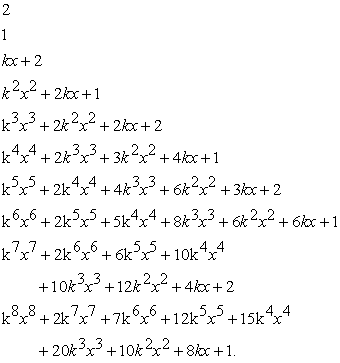(2.1)

The first few terms of the sequence {Pn} are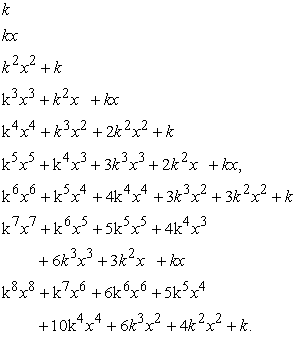(2.2)

### 3. Rising Diagonal Function

Consider the rising diagonal function of x, Un (x), un (x) for (2.1) and (2.2) respectively,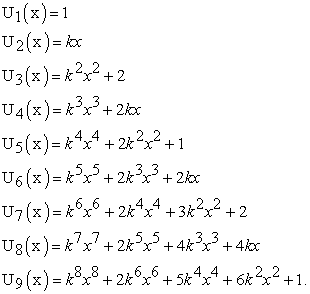(3.1)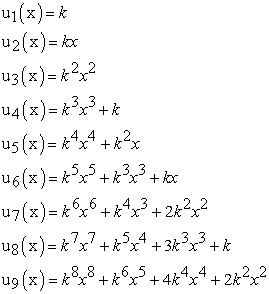(3.2)

Now, we define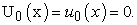(3.3)

from equation (3.1), (3.2) and (3.3) we get the following theorem:

Theorem (1). If Un (x) and un(x) are rising diagonal functions of x for sequence {Gn} and {Pn} respectively, than for,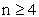(3.4)

Proof can be obtained by PMI’s method so it is obvious.

Special Case-I

If Un (x) and un(x) are rising diagonal functions of x f sequence {Gn} and {Pn} respectively, than for n=3, n=4.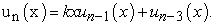(3.5)

### 4. Descending Diagonal Function

From (2.1) and (2.2), the descending diagonal function of x, Qi(x), qi(x) are(4.1)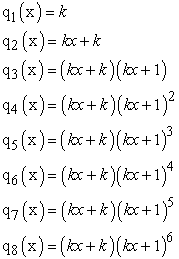(4.2)

Now, we define(4.3)

from (4.1), (4.2) and (4.3) we get for.(4.4)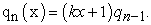(4.5)

from (4.4) and (4.5) we get the following theorem:

Theorem (2). If Qn(x) and qn(x) are descending diagonal function of x for Sequence {Gn} and {Pn} respectively, than for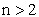.

a)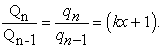b)### 5. Generating Matrix

For the sequence {Gn} defend in equation (1.1) we consider the matrix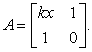(5.1)

Since, the elements of this matrix are the member of the sequence of Fibonacci Polynomials. We call this matrix as Fibonacci matrix.

Theorem (3). For sequence {Gn} we define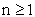,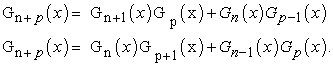Proof. For sequence {Gn}, we haveSince, determinant of matrix A is -1, therefore,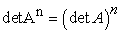(5.2)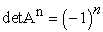(5.3)

Form equation (3.5.2) and (3.5.3), we getSince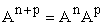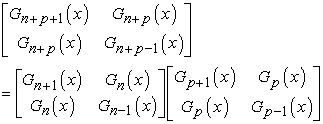After multiplying the matrices and equating the corresponding elements, we getTheorem (4). For sequence {Gn} we define,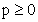Proof. For sequence {Gn}, we haveIf A is any square Matrix, then we know that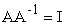(5.4)

Where I is identity matrix from equation (5.4) we get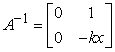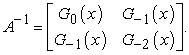By Mathematical induction, we have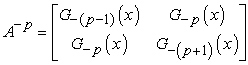Since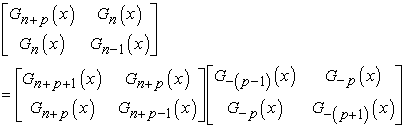After multiplying the matrices and equating the corresponding elements, we get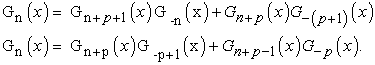### 6. Generating Matrix

For the sequence {Pn} defend in equation (1.1) we consider the matrix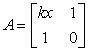(6.1)

since, the elements of this matrix are the members of the sequence of Fibonacci polynomials. We call this matrix as Fibonacci Matrix.

Theorem (5). For sequence {Pn} we define,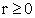Proof. For sequence {Pn}, we haveSince, determinant of matrix A is -1, there for,(6.2)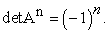By mathematical induction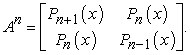(6.3)

Form equation (3.6.2) and (3.6.3), we getSinceAfter multiplying the matrices and equating the corresponding elements, we get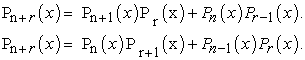Theorem (6). For sequence {Pn} we define,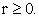Proof. For sequence {Gn}, we haveIf A is any square Matrix, then we know that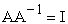(6.4)

Where I is identity matrix from equation (5.4) we getBy mathematical indication, we have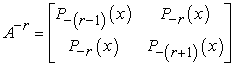Since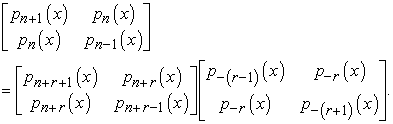After multiplying the matrices and equating the corresponding elements, we get### 7. Conclusions

In this paper Diagonal function k-Lucas Polynomials. Some basic rising diagonal function and descending diagonal function and generating matrix derived by standard method.

### Acknowledgement

We would like to thank the anonymous referees for numerous helpful suggestions.

### References

  A.F Hordam, Diagonal Function, the Fibonacci Quarterly, Vol. 16, 19-36.In article  Alexandra Lupas Guide of Fibonacci and Lucas polynomials, Octagon Mathematics Magazine Vo. 17, No.1 (1999), 2-12.In article  B.S.Porov, A note on the sum of Fibonacci and Lucas polynomials, The Fill Quarterly 1970, 428-438.In article  D.V. Jaiswal, “Some Metric Properties of a Generalized Fibonacci Sequence’’, Labdev Journal of Science and technology, India vol.11-A, No.1, 1973, 1-3.In article  Jr. V.E. Hoggatt, Fibonacci and Lucas Numbers, Houshton Mifflin Company, Borton, 1965.In article  Koshy, T. Fibonacci and Lucas number with application, Wiley, 2001.In article CrossRef  M. Catalan, An Identity for Lucas Polynomials, Fibonacci Quarterly Vol 43, No.1 2005.In article  Singh, B., S. Teeth, and Harne Diagonal Function of Fibonacci Polynomials, chh. J. Sci. Tech 2005, 97-102.In article  Vajda, S. Fibonacci and Lucas numbers and the golden section, Ellis Horwood Limited, Chi Chester, England, 1989.In article  Vorobyou, N.N., The Fibonacci number, D.C. health company, Boston, 1963.In article